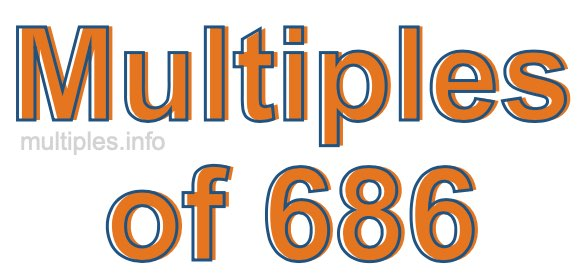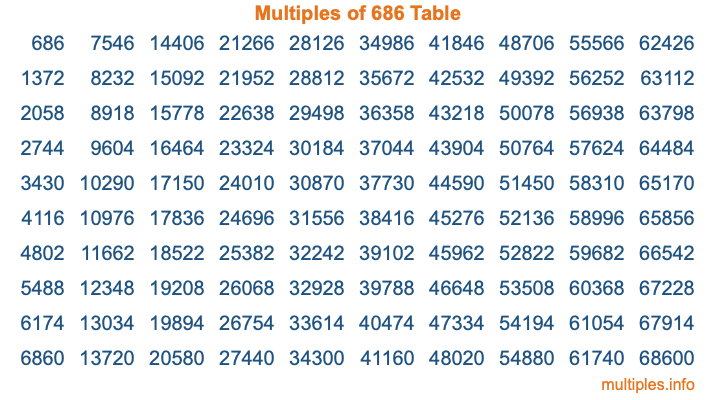Multiples of 686Welcome to the Multiples of 686 page. Here we will first teach you everything you will ever need to know about the multiples of 686, and then give you a study guide summary of everything we taught you to make sure you remember it all. Use this page to look up facts and learn information about the multiples of 686. This page will make you a multiples of six hundred eighty-six expert!

Definition of Multiples of 686
Multiples of 686 are all the numbers that when divided by 686 equal an integer. Each of the multiples of 686 are called a multiple. A multiple of 686 is created by multiplying 686 by an integer.

Therefore, to create a list of multiples of 686, you start with 1 multiplied by 686, then 2 multiplied by 686, then 3 multiplied by 686, and so on for as long as you want. Thus, the list of the first five multiples of 686 is 686, 1372, 2058, 2744, and 3430. To see a larger list of multiples of 686, see the printable image of Multiples of 686 further down on this page. We also have a category where you can choose any nth multiple of 686.

Multiples of 686 Checker
The Multiples of 686 Checker below checks to see if any number of your choice is a multiple of 686. In other words, it checks to see if there is any number (integer) that when multiplied by 686 will equal your number. To do that, we divide your number by 686. If the the quotient is an integer, then your number is a multiple of 686.

Is  a multiple of 686?

Least Common Multiple of 686 and ...
A Least Common Multiple (LCM) is the lowest multiple that two or more numbers have in common. This is also called the smallest common multiple or lowest common multiple and is useful to know when you are adding our subtracting fractions. Enter one or more numbers below (686 is already entered) to find the LCM.

Check out our LCM Calculator if you need more details about the Least Common Multiple or if you need the LCM for different numbers for adding and subtraction fractions.

nth Multiple of 686
As we stated above, 686 is the first multiple of 686, 1372 is the second multiple of 686, 2058 is the third multiple of 686, and so on. Enter a number below to find the nth multiple of 686.

th multiple of 686

Multiples of 686 vs Factors of 686
686 is a multiple of 686 and a factor of 686, but that is where the similarities end. All postive multiples of 686 are 686 or greater than 686. All positive factors of 686 are 686 or less than 686.

Below is the beginning list of multiples of 686 and the factors of 686 so you can compare:

Multiples of 686: 686, 1372, 2058, 2744, 3430, etc.

Factors of 686: 1, 2, 7, 14, 49, 98, 343, 686

As you can see, the multiples of 686 are all the numbers that you can divide by 686 to get a whole number. The factors of 686, on the other hand, are all the whole numbers that you can multiply by another whole number to get 686.

It's also interesting to note that if a number (x) is a factor of 686, then 686 will also be a multiple of that number (x).

Multiples of 686 vs Divisors of 686
The divisors of 686 are all the integers that 686 can be divided by evenly. Below is a list of the divisors of 686.

Divisors of 686: 1, 2, 7, 14, 49, 98, 343, 686

The interesting thing to note here is that if you take any multiple of 686 and divide it by a divisor of 686, you will see that the quotient is an integer.

Multiples of 686 Table
Below is an image of the first 100 multiples of 686 in a table. The table is in chronological order, column by column. The first column has the first ten multiples of 686, the second column has the next ten multiples of 686, and so on.The Multiples of 686 Table is also referred to as the 686 Times Table or Times Table of 686. You are welcome to print out our table for your studies.

Negative Multiples of 686
Although not often discussed or needed in math, it is worth mentioning that you can make a list of negative multiples of 686 by multiplying 686 by -1, then by -2, then by -3, and so on, to get the following list of negative multiples of 686:

-686, -1372, -2058, -2744, -3430, etc.

Multiples of 686 Summary
Below is a summary of important Multiples of 686 facts that we have discussed on this page. To retain the knowledge on this page, we recommend that you read through the summary and explain to yourself or a study partner why they hold true.

There are an infinite number of multiples of 686.

A multiple of 686 divided by 686 will equal a whole number.

686 divided by a factor of 686 equals a divisor of 686.

The nth multiple of 686 is n times 686.

The largest factor of 686 is equal to the first positive multiple of 686.

686 is a multiple of every factor of 686.

686 is a multiple of 686.

A multiple of 686 divided by a divisor of 686 equals an integer.

686 divided by a divisor of 686 equals a factor of 686.

Any integer times 686 will equal a multiple of 686.

Multiples of a Number
Here you can get the multiples of another number, all with the same attention to detail as we did for multiples of 686 on this page.

Multiples of
Multiples of 687
Did you find our page about multiples of six hundred eighty-six educational? Do you want more knowledge? Check out the multiples of the next number on our list!